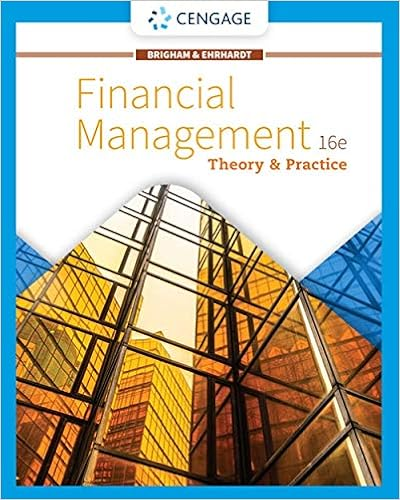# The cracker mill has a beta of 097 a dividend growth

• 59
• 94% (134) 126 out of 134 people found this document helpful

This preview shows page 28 - 31 out of 59 pages.

##### We have textbook solutions for you!
The document you are viewing contains questions related to this textbook.The document you are viewing contains questions related to this textbook.
Chapter 15 / Exercise 15-3
Financial Management: Theory & Practice
Brigham/EhrhardtExpert Verified
53. The Cracker Mill has a beta of 0.97, a dividend growth rate of 3.2 percent, a stock price of \$33 a share, and an expected annual dividend of \$1.06 per share next year. The market rate of return is 11.2 percent and the risk-free rate is 3.7 percent. What is the firm's cost of equity? A. 7.74 percentB.8.69 percentC. 9.30 percentD. 9.72 percentE. 10.01 percentRe= 0.037 + 0.97(0.112 - 0.037) = 0.10975Re= (\$1.06/\$33) + 0.032 = 0.064121Average Re= (0.10975 + 0.064121)/2 = 8.69 percent
AACSB: AnalyticBloom's: AnalysisDifficulty: BasicLearning Objective: 12-01 Determine a firm's cost of equity capitalSection: 12.2Topic: Cost of equity54. The market rate of return is 14.8 percent and the risk-free rate is 4.45 percent. Galaxy Co. has 54 percent more systematic risk than the overall market and has a dividend growth rate of 5.5 percent. The firm's stock is currently selling for \$39 a share and has a dividend yield of 3.6 percent. What is the firm's cost of equity?
AACSB: AnalyticBloom's: AnalysisDifficulty: BasicLearning Objective: 12-01 Determine a firm's cost of equity capitalSection: 12.2Topic: Cost of equity12-28
##### We have textbook solutions for you!
The document you are viewing contains questions related to this textbook.The document you are viewing contains questions related to this textbook.
Chapter 15 / Exercise 15-3
Financial Management: Theory & Practice
Brigham/EhrhardtExpert Verified
Chapter 12 - Cost of Capital55. Appalachian Mountain Goods has paid increasing dividends of \$.0.12, \$0.18, \$0.20, and \$0.25 a share over the past four years, respectively. The firm estimates that future increases in its dividends will be equal to the arithmetic average growth rate over these past four years. The stock is currently selling for \$12.60 a share. The risk-free rate is 3.2 percent and the market risk premium is 9.1 percent. What is the cost of equity for this firm if its beta is 1.26?
AACSB: AnalyticBloom's: AnalysisDifficulty: BasicLearning Objective: 12-01 Determine a firm's cost of equity capitalSection: 12.2Topic: Cost of equity12-29
Chapter 12 - Cost of Capital56. Winter Wear, Inc. has 6 percent bonds outstanding that mature in 13 years. The bonds pay interest semiannually and have a face value of \$1,000. Currently, the bonds are selling for \$993 each. What is the firm's pre-tax cost of debt?
AACSB: AnalyticBloom's: AnalysisDifficulty: BasicLearning Objective: 12-02 Determine a firm's cost of debtSection: 12.3Topic: Cost of debt
•••